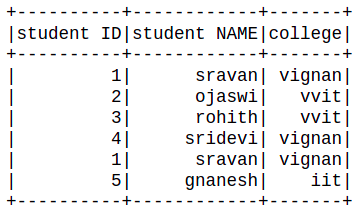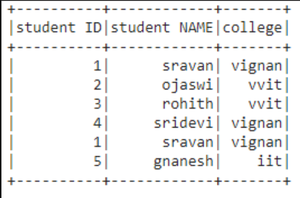# How to select last row and access PySpark dataframe by index ?

In this article, we will discuss how to select the last row and access pyspark dataframe by index.

Creating dataframe for demonstration:

## Python3

 `# importing module` `import` `pyspark`   `# importing sparksession from pyspark.sql module` `from` `pyspark.sql ``import` `SparkSession`   `# creating sparksession and giving an app name` `spark ``=` `SparkSession.builder.appName(``'sparkdf'``).getOrCreate()`   `# list  of students  data ` `data ``=` `[[``"1"``,``"sravan"``,``"vignan"``],` `       ``[``"2"``,``"ojaswi"``,``"vvit"``],` `       ``[``"3"``,``"rohith"``,``"vvit"``],` `       ``[``"4"``,``"sridevi"``,``"vignan"``],` `       ``[``"1"``,``"sravan"``,``"vignan"``], ` `       ``[``"5"``,``"gnanesh"``,``"iit"``]]`   `# specify column names` `columns ``=` `[``'student ID'``,``'student NAME'``,``'college'``]`   `# creating a dataframe from the lists of data` `dataframe ``=` `spark.createDataFrame(data,columns)`   `# show dataframe` `dataframe.show()`

Output:### Select last row from dataframe

Example 1: Using tail() function.

This function is used to access the last row of the dataframe

Syntax: dataframe.tail(n)

where

1. n is the number of rows to be selected from the last.
2. dataframe is the input dataframe

We can use n = 1 to select only last row.

Example 1: Selecting last row.

## Python3

 `# access last row of the dataframe` `dataframe.tail(``1``)`

Output:

[Row(student ID=’5′, student NAME=’gnanesh’, college=’iit’)]

Example 2: Python program to access last N rows.

## Python3

 `# access last 5 rows of the` `# dataframe` `dataframe.tail(``5``)`

Output:

```[Row(student ID='2', student NAME='ojaswi', college='vvit'),
Row(student ID='3', student NAME='rohith', college='vvit'),
Row(student ID='4', student NAME='sridevi', college='vignan'),
Row(student ID='1', student NAME='sravan', college='vignan'),
Row(student ID='5', student NAME='gnanesh', college='iit')]```

### Access the dataframe by column index

Here we are going to select the dataframe based on the column number. For selecting a specific column by using column number in the pyspark dataframe, we are using select() function

Syntax: dataframe.select(dataframe.columns[column_number]).show()

where,

1. dataframe is the dataframe name
2. dataframe.columns[]: is the method which can take column number as an input and select those column
3. show() function is used to display the selected column

Example 1: Python program to access column based on column number

## Python3

 `# select column with column number 1` `dataframe.select(dataframe.columns[``1``]).show()`

Output:

```+------------+
|student NAME|
+------------+
|      sravan|
|      ojaswi|
|      rohith|
|     sridevi|
|      sravan|
|     gnanesh|
+------------+```

Example 2: Accessing multiple columns based on column number, here we are going to select multiple columns by using the slice operator, It can access upto n columns

Syntax: dataframe.select(dataframe.columns[column_start:column_end]).show()

where: column_start is the starting index and column_end is the ending index.

## Python3

 `# select column with column number slice` `# operator` `dataframe.select(dataframe.columns[``0``:``3``]).show()`

Output:Whether you're preparing for your first job interview or aiming to upskill in this ever-evolving tech landscape, GeeksforGeeks Courses are your key to success. We provide top-quality content at affordable prices, all geared towards accelerating your growth in a time-bound manner. Join the millions we've already empowered, and we're here to do the same for you. Don't miss out - check it out now!